Home > PC > Chapter 11 > Lesson 11.2.1 > Problem11-74

11-74.
1. Find the following limits, rationalizing the numerator where necessary. Homework Help ✎

2. Example: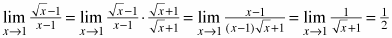1.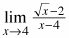2.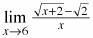3.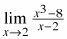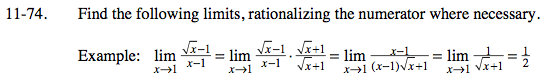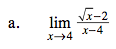After rationalizing the numerator, did you get:

$\lim_{ \textit{x} \to 4 }\frac{\textit{x}-4}{(\textit{x}-4)(\sqrt{\textit{x}}+2)}$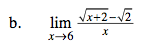Is it necessary to rationalize the numerator in this case?
What happens when you just use substitution?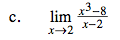Recall the cubic factoring formula:

$\textit{a}^3-\textit{b}^3=(\textit{a}-\textit{b})(\textit{a}^2+\textit{ab}+\textit{b}^2)$